# ISEE Middle Level Math : How to find the decimal equivalent of a fraction

## Example Questions

← Previous 1 3 4 5 6 7 8 9 18 19

### Example Question #1 : How To Find The Decimal Equivalent Of A Fraction

Rewrite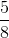as a decimal.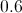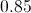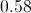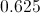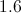Explanation:

Divideby; the result is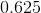.

You may also recognize thatis equal to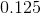, andwill be equal totimes this value.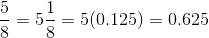### Example Question #2 : How To Find The Decimal Equivalent Of A Fraction

Write the decimal equivalent of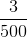.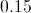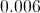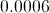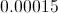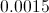Explanation: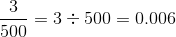### Example Question #3 : How To Find The Decimal Equivalent Of A Fraction

How do you write 0.125 as a fraction?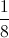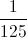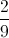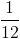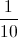Explanation: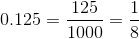### Example Question #4 : How To Find The Decimal Equivalent Of A Fraction

Express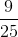as a decimal.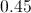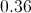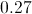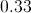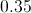Explanation:

Divide the numerator by the denominator: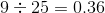### Example Question #5 : How To Find The Decimal Equivalent Of A Fraction

Express 0.36 as a fraction in simplest form.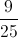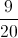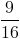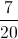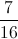Explanation:

Write the expression, without the decimal point (36), over the apporpriate power of 10 (100, since the last digit falls in the hundredths place). Then reduce: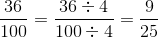### Example Question #6 : How To Find The Decimal Equivalent Of A Fraction

Which is equivalent to this fraction?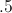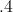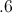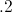Explanation:

Divide: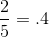### Example Question #7 : How To Find The Decimal Equivalent Of A Fraction

Write as a decimal: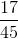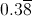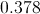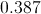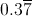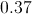Explanation:

Divide 17 by 45, adding a decimal point and zeroes as needed: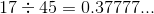The 7 repeats infinitely. The decimal is therefore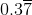.

### Example Question #8 : How To Find The Decimal Equivalent Of A Fraction

Write as a fraction in lowest terms: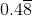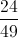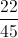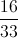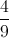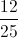Explanation:

We can use algebra to find the fraction by setting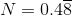. Then: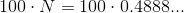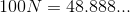and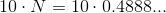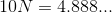Subtract as follows: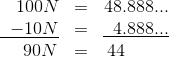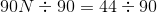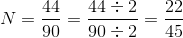### Example Question #9 : How To Find The Decimal Equivalent Of A Fraction

Convert 0.017 into a fraction.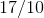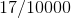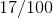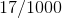Explanation:

0.017 is equal to 17 thousandths (because it extends 3 decimal places), therefore: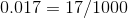### Example Question #10 : How To Find The Decimal Equivalent Of A Fraction

Write as a decimal: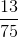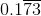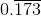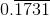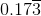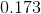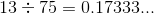The 3 repeats infinitely, so we can rewrite this as.